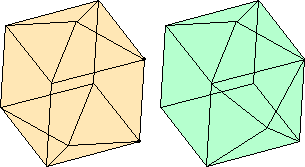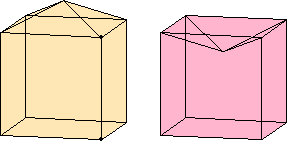# Definition 9

Similar solid figures are those contained by similar planes equal in multitude.

# Definition 10

Equal and similar solid figures are those contained by similar planes equal in multitude and magnitude.

## Guide

While definition 9 defines similar solid figures, definition 10 describes what is commonly called congruent solid figures. Euclid uses “equal and similar” plane figures for congruent plane figures in these later books, but that could not be done for plane figures before Book VI where similarity of plane figures was defined.

These definitions are incomplete as was VI.Def.1 for similar rectilinear figures. The notion of similarity for plane figures implicitly assumed a correspondence of consecutive vertices and sides. This notion of similar solid figures assumes a correspondence of of adjacent edges and faces.

 Different solid figures can sometimes be constructed with the same faces but with different adjacencies. For example, there are two distinct ways to attach two pyramids to two of the square faces of a cube. They could be attached to opposite faces of the cube or to adjacent faces of the cube. The resulting solids both have four remaining square faces and eight new triangular faces, but the positioning of the squares and triangles is different. Heron’s definition of similar solid figures, “those which are contained by equal and similarly situated planes, equal in number and magnitude,” is a bit more explicit than Euclid’s.It is also apparent that Euclid did not consider the possibility of concave solids and the problems they cause his definition, problems that Simson noticed. Take a cube and first add a pyramid on the outside of one square face, and second subtract the same pyramid from the inside of a square face. The resulting two solids both have five square faces and four triangular faces, and the adjacencies are the same, but they are very different solids. To eliminate this problem, further conditions must be made on the definition of similar solids. For instance, conditions on dihedral angles between faces, or conditions on distances between all corresponding pairs of vertices.